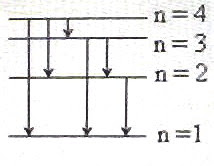## Pages

`“Life is like riding a bicycle.  To keep your balance you must keep moving.”–Albert Einstein`

## Monday, February 25, 2008

### AP Physics B– Multiple Choice Questions (for practice) on Atomic Physics and Quantum Effects

As promised in the post dated 23-2-08, I give below some typical multiple choice questions (MCQ) for practice:

(1) Five photons have the following energy values. Which one represents the visible light photon?

(a) 24.8 eV

(b)12.4 eV

(c) 6.2 eV

(d) 2.48 eV

(e) 1.24 eV

Photons in the visible region have wave length range from 4000 Ǻ to 7000 Ǻ (approximately). The product of the energy in eV and the wave length in Angstrom in the case of any photon is 12400 (very nearly).

The energy of the 4000 Ǻ photon in electron volt is 12400/4000 = 3.1 eV.

The energy of the 7000 Ǻ photon in electron volt is 12400/7000 = 1.77 eV.

Therefore, the photon in the visible region is given in option (d).

If you were asked to calculate the wave length of the photon of energy 2.48 eV, you will have

λ = 12400/2.48 = 5000 Ǻ

[You can calculate the wave length using the relation E = hc/λ where E is the energy in joule, h is Planck’s constant, c is the speed of light and λ is the wave length (in metre). In the above problem E = 2.48×1.6×10–19 joule (on converting the energy in eV into joule), c = 3×108 ms–1and h = 6.6×10–34 Js. But this is time consuming].

(2) A laser source gives light output of power P. If the wave length of the laser is λ, the number of photons emitted in a time t in terms of the given parameters and fundamental constants is

(a) Pλt/hc

(b) Phct/λ

(c) Pht/λc

(d) Pλ/hc

(e) Pct/hλ

The energy of a photon is hν = hc/λ where h is Planck’s constant, ν is the frequency of light, c is the speed of light and λ is the wave length.

Number of photons emitted per second = P/(hc/λ) = Pλ/hc.

Therefore, total number of photons emitted in time t = Pλt/hc.

(3) When electromagnetic radiations of wave length λ is incident on a photosensitive surface, the kinetic energy of the photoelectrons emitted from the surface is 2 eV. When the wave length of the incident radiations is 2λ, the kinetic energy of the photoelectrons emitted from the surface is 0.5 eV. The threshold wave length (maximum wave length) for photoelectric emission from the surface is

(a) λ/2

(b) λ

(c) 3λ/2

(d) 2λ

(e)

From Einstein’s equation, we have for the two cases

hc/λ = hc/λ0 + 2 eV and

hc/2λ = hc/λ0 + 0.5 eV

where h is Planck’s constant, c is the speed of light and λ0 is the threshold wave length. We have expressed the kinetic energy in electron volt itself for convenience, with the understanding that all terms are in electron volt.

Multiplying the second equation by 4 and subtracting the first equation from it, we obtain

hc/λ = 3hc/λ0 from which λ0 = 3λ.

(4) According to the Bohr model, electrons of quantum number n = 4 in excited hydrogen atoms can undergo transitions to lower energy states in different ways and give rise to photons of discrete frequencies. How many discrete frequencies are possible in this case?

(a) 3

(b) 4(c) 6

(d) 8

(e) 12The possible transitions are shown in the adjoining figure. You will see 6 different transitions. Therefore, there will be six discrete frequencies.

You need not draw an energy level diagram to get the answer for similar questions. {If n is large, the method will be difficult). Remember that the possible number of transitions is n(n – 1)/2.

(5) Singly ionized helium (He+) atom is hydrogen like in the sense that a solitary electron revolves around a positively charged nucleus. If the energy of this electron in its first orbit (n = 1) is –54.4 eV, what will be its energy in the first excited state?

(a) –108.8 eV

(b) –27.2 eV

(c) 13.6 eV

(d) 6.8 eV

(e) 3.4 eV

First excited state means the electron is in the second orbit (n = 2).

For a hydrogen like atom, the energy of the electron in the orbit of quantum number ‘n’ is inversely proportional to n2. Since the energy in the first orbit is –54.4 eV, the energy in the second orbit will be –54.4/22 eV = –13.6 eV

Few more questions will be posted from this section in the next post.

You will find a useful post on hydrogen like atoms at physicsplus